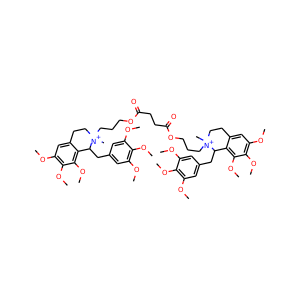877-202-0205     support@chemchart.com     @chemchartDoxacurium
Weight 1035.238 g/mol C56H78N2O16+2 16 0 4 27

# Doxacurium (106819-53-8, 133814-18-3)

"What do you need help with?"### Alternate Names

• SMILES
C[N+]1(CCC2=CC(=C(C(=C2C1CC3=CC(=C(C(=C3)OC)OC)OC)OC)OC)OC)CCCOC(=O)CCC(=O)OCCC[N+]4(CCC5=CC(=C(C(=C5C4CC6=CC(=C(C(=C6)OC)OC)OC)OC)OC)OC)C
• InChIKey
GBLRQXKSCRCLBZ-UHFFFAOYSA-N